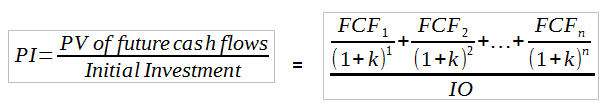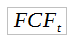# Profitability Index Assignment Help

## What is profitability index?

A profitability index (PI) is the relationship between the costs and benefits of a proposed project. It is calculated by dividing the present value of the project’s future cash flows by the initial amount invested.

PI, also known as benefit-cost ratio is calculated as follows:In the equation aboverepresents the annual free cash flow in time period t and can be either positive or negative, k is the required rate of return (cost of capital), IO is the initial investment and n is the expected life of the project. A PI greater than 1.0 indicates that profitability is positive, while a PI of less than 1.0 indicates that the project will lose money.

As values on the PI increase, the proposed project becomes more financially appealing. The PI rule is a variation of the net present value (NPV) rule. Usually, if NPV is positive, the PI would be greater than 1. If NPV is negative, the PI would be below 1. However, the PI differs from NPV in that it ignores the size of the investment and provides no indication of the amount of the actual cash flows. Basically the PI is a project’s NPV turned into a percentage rate. It may seem a little bit confusing but if you have problems our finance homework service can help.

Our service can provide assistance with any finance topic at any academic level and that includes profitability index assignment help and risk & return assignment help. As with many finance topics there are many concepts you must be familiar with to tackle a profitability index assignment successfully. Present value, future cash flows and required rate of return are some of the things you must know about when you are dealing with the benefit-cost ratio. Our service will you understand every concept involved and walk you through the PI equation providing a detailed explanation of how to do each step and why it is necessary. Our help isn’t limited to finance homework assignments. We can also assist with test preparation, research papers and individual finance tutoring for especially difficult areas or even for the entire course should you need it.